Skip to main content

# Statistics review 4: Sample size calculations

## Abstract

The present review introduces the notion of statistical power and the hazard of under-powered studies. The problem of how to calculate an ideal sample size is also discussed within the context of factors that affect power, and specific methods for the calculation of sample size are presented for two common scenarios, along with extensions to the simplest case.

## Introduction

Previous reviews in this series introduced confidence intervals and P values. Both of these have been shown to depend strongly on the size of the study sample in question, with larger samples generally resulting in narrower confidence intervals and smaller P values. The question of how large a study should ideally be is therefore an important one, but it is all too often neglected in practice. The present review provides some simple guidelines on how best to choose an appropriate sample size.

Research studies are conducted with many different aims in mind. A study may be conducted to establish the difference, or conversely the similarity, between two groups defined in terms of a particular risk factor or treatment regimen. Alternatively, it may be conducted to estimate some quantity, for example the prevalence of disease, in a specified population with a given degree of precision. Regardless of the motivation for the study, it is essential that it be of an appropriate size to achieve its aims. The most common aim is probably that of determining some difference between two groups, and it is this scenario that will be used as the basis for the remainder of the present review. However, the ideas underlying the methods described are equally applicable to all settings.

## Power

The difference between two groups in a study will usually be explored in terms of an estimate of effect, appropriate confidence interval and P value. The confidence interval indicates the likely range of values for the true effect in the population, while the P value determines how likely it is that the observed effect in the sample is due to chance. A related quantity is the statistical power of the study. Put simply, this is the probability of correctly identifying a difference between the two groups in the study sample when one genuinely exists in the populations from which the samples were drawn.

The ideal study for the researcher is one in which the power is high. This means that the study has a high chance of detecting a difference between groups if one exists; consequently, if the study demonstrates no difference between groups the researcher can be reasonably confident in concluding that none exists in reality. The power of a study depends on several factors (see below), but as a general rule higher power is achieved by increasing the sample size.

It is important to be aware of this because all too often studies are reported that are simply too small to have adequate power to detect the hypothesized effect. In other words, even when a difference exists in reality it may be that too few study subjects have been recruited. The result of this is that P values are higher and confidence intervals wider than would be the case in a larger study, and the erroneous conclusion may be drawn that there is no difference between the groups. This phenomenon is well summed up in the phrase, 'absence of evidence is not evidence of absence'. In other words, an apparently null result that shows no difference between groups may simply be due to lack of statistical power, making it extremely unlikely that a true difference will be correctly identified.

Given the importance of this issue, it is surprising how often researchers fail to perform any systematic sample size calculations before embarking on a study. Instead, it is not uncommon for decisions of this sort to be made arbitrarily on the basis of convenience, available resources, or the number of easily available subjects. A study by Moher and coworkers  reviewed 383 randomized controlled trials published in three journals (Journal of the American Medical Association, Lancet and New England Journal of Medicine) in order to examine the level of statistical power in published trials with null results. Out of 102 null trials, those investigators found that only 36% had 80% power to detect a relative difference of 50% between groups and only 16% had 80% power to detect a more modest 25% relative difference. (Note that a smaller difference is more difficult to detect and requires a larger sample size; see below for details.) In addition, only 32% of null trials reported any sample size calculations in the published report. The situation is slowly improving, and many grant giving bodies now require sample size calculations to be provided at the application stage. Many under-powered studies continue to be published, however, and it is important for readers to be aware of the problem.

Finally, although the most common criticism of the size, and hence the power, of a study is that it is too low, it is also worth noting the consequences of having a study that is too large. As well as being a waste of resources, recruiting an excessive number of participants may be unethical, particularly in a randomized controlled trial where an unnecessary doubling of the sample size may result in twice as many patients receiving placebo or potentially inferior care, as is necessary to establish the worth of the new therapy under consideration.

## Factors that affect sample size calculations

It is important to consider the probable size of study that will be required to achieve the study aims at the design stage. The calculation of an appropriate sample size relies on a subjective choice of certain factors and sometimes crude estimates of others, and may as a result seem rather artificial. However, it is at worst a well educated guess, and is considerably more useful than a completely arbitrary choice. There are three main factors that must be considered in the calculation of an appropriate sample size, as summarized in Table 1. The choice of each of these factors impacts on the final sample size, and the skill is in combining realistic values for each of these in order to achieve an attainable sample size. The ultimate aim is to conduct a study that is large enough to ensure that an effect of the size expected, if it exists, is sufficiently likely to be identified.

Although, as described in Statistics review 3, it is generally bad practice to choose a cutoff for statistical 'significance' based on P values, it is a convenient approach in the calculation of sample size. A conservative cutoff for significance, as indicated by a small P value, will reduce the risk of incorrectly interpreting a chance finding as genuine. However, in practice this caution is reflected in the need for a larger sample size in order to obtain a sufficiently small P value. Similarly, a study with high statistical power will, by definition, make identification of any difference relatively easy, but this can only be achieved in a sufficiently large study. In practice there are conventional choices for both of these factors; the P value for significance is most commonly set at 0.05, and power will generally be somewhere between 80% and 95%, depending on the resulting sample size.

The remaining factor that must be considered is the size of the effect to be detected. However, estimation of this quantity is not always straightforward. It is a crucial factor, with a small effect requiring a large sample and vice versa, and careful consideration should be given to the choice of value. Ideally, the size of the effect will be based on clinical judgement. It should be large enough to be clinically important but not so large that it is implausible. It may be tempting to err on the side of caution and to choose a small effect; this may well cover all important clinical scenarios but will be at the cost of substantially (and potentially unnecessarily) increasing the sample size. Alternatively, an optimistic estimate of the probable impact of some new therapy may result in a small calculated sample size, but if the true effect is less impressive than expected then the resulting study will be under-powered, and a smaller but still important effect may be missed.

Once these three factors have been established, there are tabulated values  and formulae available for calculating the required sample size. Certain outcomes and more complex study designs may require further information, and calculation of the required sample size is best left to someone with appropriate expertise. However, specific methods for two common situations are detailed in the following sections.

Note that the sample sizes obtained from these methods are intended as approximate guides rather than exact numbers. In other words a calculation indicating a sample size of 100 will generally rule out the need for a study of size 500 but not one of 110; a sample size of 187 can be usefully rounded up to 200, and so on. In addition, the results of a sample size calculation are entirely dependent on estimates of effect, power and significance, as discussed above. Thus, a range of values should be incorporated into any good investigation in order to give a range of suitable sample sizes rather than a single 'magic' number.

## Sample size calculation for a difference in means (equal sized groups)

Let us start with the simplest case of two equal sized groups. A recently published trial  considered the effect of early goal-directed versus traditional therapy in patients with severe sepsis or septic shock. In addition to mortality (the primary outcome on which the study was originally powered), the investigators also considered a number of secondary outcomes, including mean arterial pressure 6 hours after the start of therapy. Mean arterial pressure was 95 and 81 mmHg in the groups treated with early goal-directed and traditional therapy, respectively, corresponding to a difference of 14 mmHg.

The first step in calculating a sample size for comparing means is to consider this difference in the context of the inherent variability in mean arterial pressure. If the means are based on measurements with a high degree of variation, for example with a standard deviation of 40 mmHg, then a difference of 14 mmHg reflects a relatively small treatment effect compared with the natural spread of the data, and may well be unremarkable. Conversely, if the standard deviation is extremely small, say 3 mmHg, then an absolute difference of 14 mmHg is considerably more important. The target difference is therefore expressed in terms of the standard deviation, known as the standardized difference, and is defined as follows: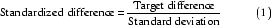In practice the standard deviation is unlikely to be known in advance, but it may be possible to estimate it from other similar studies in comparable populations, or perhaps from a pilot study. Again, it is important that this quantity is estimated realistically because an overly conservative estimate at the design stage may ultimately result in an under-powered study.

In the current example the standard deviation for the mean arterial pressure was approximately 18 mmHg, so the standardized difference to be detected, calculated using equation 1, was 14/18 = 0.78. There are various formulae and tabulated values available for calculating the desired sample size in this situation, but a very straightforward approach is provided by Altman  in the form of the nomogram shown in Fig. 1 .Figure 1

The left-hand axis in Fig. 1 shows the standardized difference (as calculated using Eqn 1, above), while the right-hand axis shows the associated power of the study. The total sample size required to detect the standardized difference with the required power is obtained by drawing a straight line between the power on the right-hand axis and the standardized difference on the left-hand axis. The intersection of this line with the upper part of the nomogram gives the sample size required to detect the difference with a P value of 0.05, whereas the intersection with the lower part gives the sample size for a P value of 0.01. Fig. 2 shows the required sample sizes for a standardized difference of 0.78 and desired power of 0.8, or 80%. The total sample size for a trial that is capable of detecting a 0.78 standardized difference with 80% power using a cutoff for statistical significance of 0.05 is approximately 52; in other words, 26 participants would be required in each arm of the study. If the cutoff for statistical significance were 0.01 rather than 0.05 then a total of approximately 74 participants (37 in each arm) would be required.Figure 2

The effect of changing from 80% to 95% power is shown in Fig. 3. The sample sizes required to detect the same standardized difference of 0.78 are approximately 86 (43 per arm) and 116 (58 per arm) for P values of 0.05 and 0.01, respectively.Figure 3

The nomogram provides a quick and easy method for determining sample size. An alternative approach that may offer more flexibility is to use a specific sample size formula. An appropriate formula for comparing means in two groups of equal size is as follows: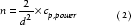where n is the number of subjects required in each group, d is the standardized difference and cp,power is a constant defined by the values chosen for the P value and power. Some commonly used values for cp,power are given in Table 2. The number of participants required in each arm of a trial to detect a standardized difference of 0.78 with 80% power using a cutoff for statistical significance of 0.05 is as follows: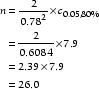Thus, 26 participants are required in each arm of the trial, which agrees with the estimate provided by the nomogram.

## Sample size calculation for a difference in proportions (equal sized groups)

A similar approach can be used to calculate the sample size required to compare proportions in two equally sized groups. In this case the standardized difference is given by the following equation:where p1 and p2 are the proportions in the two groups and= (p1 + p2)/2 is the mean of the two values. Once the standardized difference has been calculated, the nomogram shown in Fig. 1 can be used in exactly the same way to determine the required sample size.

As an example, consider the recently published Acute Respiratory Distress Syndrome Network trial of low versus traditional tidal volume ventilation in patients with acute lung injury and acute respiratory distress syndrome . Mortality rates in the low and traditional volume groups were 31% and 40%, respectively, corresponding to a reduction of 9% in the low volume group. What sample size would be required to detect this difference with 90% power using a cutoff for statistical significance of 0.05? The mean of the two proportions in this case is 35.5% and the standardized difference is therefore as follows (calculated using Eqn 3).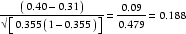Fig. 4 shows the required sample size, estimated using the nomogram to be approximately 1200 in total (i.e. 600 in each arm).Figure 4

Again, there is a formula that can be used directly in these circumstances. Comparison of proportions p1 and p2 in two equally sized groups requires the following equation: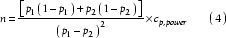where n is the number of subjects required in each group and cp,power is as defined in Table 2. Returning to the example of the Acute Respiratory Distress Syndrome Network trial, the formula indicates that the following number of patients would be required in each arm.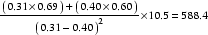This estimate is in accord with that obtained from the nomogram.

## Calculating power

The nomogram can also be used retrospectively in much the same way to calculate the power of a published study. The Acute Respiratory Distress Syndrome Network trial stopped after enrolling 861 patients. What is the power of the published study to detect a standardized difference in mortality of 0.188, assuming a cutoff for statistical significance of 0.05?

The patients were randomized into two approximately equal sized groups (432 and 429 receiving low and traditional tidal volumes, respectively), so the nomogram can be used directly to estimate the power. (For details on how to handle unequally sized groups, see below.) The process is similar to that for determining sample size, with a straight line drawn between the standardized difference and the sample size extended to show the power of the study. This is shown for the current example in Fig. 5, in which a (solid) line is drawn between a standardized difference of 0.188 and an approximate sample size of 861, and is extended (dashed line) to indicate a power of around 79%.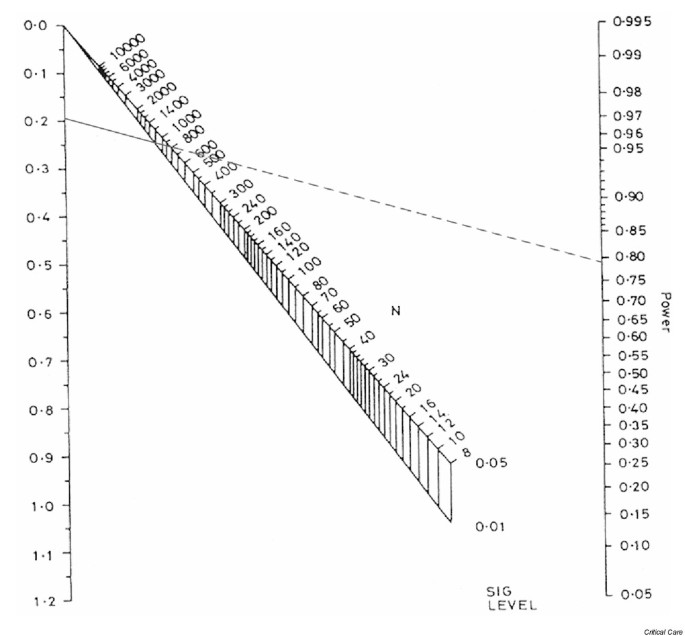Figure 5

It is also possible to use the nomogram in this way when financial or logistical constraints mean that the ideal sample size cannot be achieved. In this situation, use of the nomogram may enable the investigator to establish what power might be achieved in practice and to judge whether the loss of power is sufficiently modest to warrant continuing with the study.

As an additional example, consider data from a published trial of the effect of prone positioning on the survival of patients with acute respiratory failure . That study recruited a total of 304 patients into the trial and randomized 152 to conventional (supine) positioning and 152 to a prone position for 6 h or more per day. The trial found that patients placed in a prone position had improved oxygenation but that this was not reflected in any significant reduction in survival at 10 days (the primary end-point).

Mortality rates at 10 days were 21% and 25% in the prone and supine groups, respectively. Using equation 3, this corresponds to a standardized difference of the following: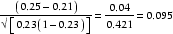This is comparatively modest and is therefore likely to require a large sample size to detect such a difference in mortality with any confidence. Fig. 6 shows the appropriate nomogram, which indicates that the published study had only approximately 13% power to detect a difference of this size using a cutoff for statistical significance of 0.05. In other words even if, in reality, placing patients in a prone position resulted in an important 4% reduction in mortality, a trial of 304 patients would be unlikely to detect it in practice. It would therefore be dangerous to conclude that positioning has no effect on mortality without corroborating evidence from another, larger trial. A trial to detect a 4% reduction in mortality with 80% power would require a total sample size of around 3500 (i.e. approximately 1745 patients in each arm). However, a sample size of this magnitude may well be impractical. In addition to being dramatically under-powered, that study has been criticized for a number of other methodological/design failings [8,9]. Sadly, despite the enormous effort expended, no reliable conclusions regarding the efficacy of prone positioning in acute respiratory distress syndrome can be drawn from the trial.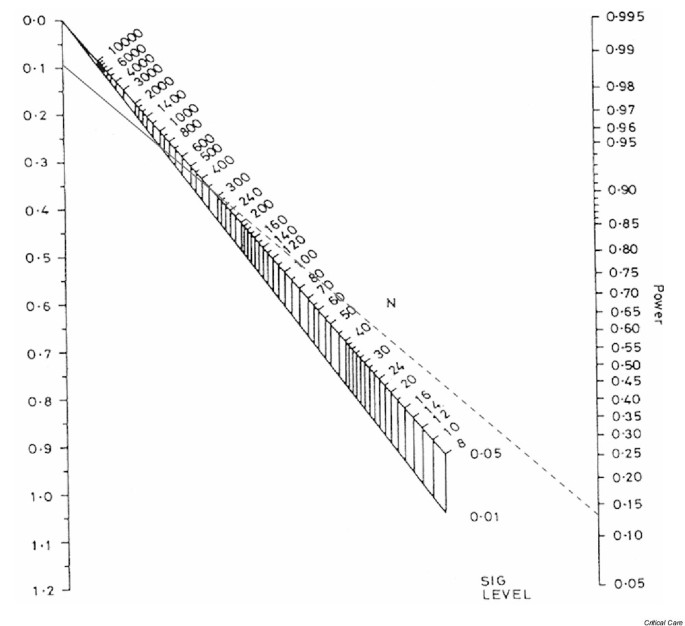Figure 6

## Unequal sized groups

The methods described above assume that comparison is to be made across two equal sized groups. However, this may not always be the case in practice, for example in an observational study or in a randomized controlled trial with unequal randomization. In this case it is possible to adjust the numbers to reflect this inequality. The first step is to calculate the total sample size (across both groups) assuming that the groups are equal sized (as described above). This total sample size (N) can then be adjusted according to the actual ratio of the two groups (k) with the revised total sample size (N') equal to the following: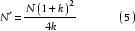and the individual sample sizes in each of the two groups are N'/(1 + k) and kN'/(1 + k).

Returning to the example of the Acute Respiratory Distress Syndrome Network trial, suppose that twice as many patients were to be randomized to the low tidal volume group as to the traditional group, and that this inequality is to be reflected in the study size. Fig. 4 indicates that a total of 1200 patients would be required to detect a standardized difference of 0.188 with 90% power. In order to account for the ratio of low to traditional volume patients (k = 2), the following number of patients would be required.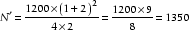This comprises 1350/3 = 450 patients randomized to traditional care and (2 × 1350)/3 = 900 to low tidal volume ventilation.

## Withdrawals, missing data and losses to follow up

Any sample size calculation is based on the total number of subjects who are needed in the final study. In practice, eligible subjects will not always be willing to take part and it will be necessary to approach more subjects than are needed in the first instance. In addition, even in the very best designed and conducted studies it is unusual to finish with a dataset in which complete data are available in a usable format for every subject. Subjects may fail or refuse to give valid responses to particular questions, physical measurements may suffer from technical problems, and in studies involving follow up (e.g. trials or cohort studies) there will always be some degree of attrition. It may therefore be necessary to calculate the number of subjects that need to be approached in order to achieve the final desired sample size.

More formally, suppose a total of N subjects is required in the final study but a proportion (q) are expected to refuse to participate or to drop out before the study ends. In this case the following total number of subjects would have to be approached at the outset to ensure that the final sample size is achieved: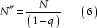For example, suppose that 10% of subjects approached in the early goal-directed therapy trial described above are expected to refuse to participate. Then, considering the effect on mean arterial pressure and assuming a P for statistical significance of 0.05 and 80% power, the following total number of eligible subjects would have to be approached in the first instance: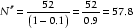Thus, around 58 eligible subjects (approximately 29 in each arm) would have to be approached in order to ensure the required final sample size of 52 is achieved.

As with other aspects of sample size calculations, the proportion of eligible subjects who will refuse to participate or provide inadequate information will be unknown at the onset of the study. However, good estimates will often be possible using information from similar studies in comparable populations or from an appropriate pilot study. Note that it is particularly important to account for nonparticipation in the costing of studies in which initial recruitment costs are likely to be high.

## Key messages

Studies must be adequately powered to achieve their aims, and appropriate sample size calculations should be carried out at the design stage of any study.

Estimation of the expected size of effect can be difficult and should, wherever possible, be based on existing evidence and clinical expertise. It is important that any estimates be large enough to be clinically important while also remaining plausible.

Many apparently null studies may be under-powered rather than genuinely demonstrating no difference between groups; absence of evidence is not evidence of absence.

## References

1. 1.

Moher D, Dulberg CS, Wells GA: Statistical power, sample size, and their reporting in randomized controlled trials. JAMA. 1994, 272: 122-124. 10.1001/jama.272.2.122.

2. 2.

Machin D, Campbell MJ, Fayers P, Pinol A: Sample Size Tables for Clinical Studies. Oxford, UK: Blackwell Science Ltd;. 1987

3. 3.

Rivers E, Nguyen B, Havstad S, Ressler J, Muzzin A, Knoblich B, Peterson E, Tomlanovich M: Early goal-directed therapy in the treatment of severe sepsis and septic shock. N Engl J Med. 2001, 345: 1368-1377. 10.1056/NEJMoa010307.

4. 4.

Altman DG: Practical Statistics for Medical Research. London, UK; Chapman & Hall;. 1991

5. 5.

Altman DG: How large a sample?. In: Statistics in Practice. Edited by: Gore SM, Altman DG. 1982, London, UK: British Medical Association

6. 6.

Anonymous: Ventilation with lower tidal volumes as compared with traditional tidal volumes for acute lung injury and the acute respiratory distress syndrome. The Acute Respiratory Distress Syndrome Network. N Engl J Med. 1996, 342: 1301-1308.

7. 7.

Gattinoni L, Tognoni G, Pesenti A, Taccone P, Mascheroni D, Labarta V, Malacrida R, Di Giulio P, Fumagalli R, Pelosi P, Brazzi L, Latini R, Prone-Supine Study Group: Effect of prone positioning on the survival of patients with acute respiratory failure. N Engl J Med. 2001, 345: 568-573. 10.1056/NEJMoa010043.

8. 8.

Zijlstra JG, Ligtenberg JJ, van der Werf TS: Prone positioning of patients with acute respiratory failure. N Engl J Med. 2002, 346: 295-297. 10.1056/NEJM200201243460417.

9. 9.

Slutsky AS: The acute respiratory distress syndrome, mechanical ventilation, and the prone position. N Engl J Med. 2001, 345: 610-612. 10.1056/NEJM200108233450811.

Download references

Authors

None declared.

## Rights and permissions

Reprints and Permissions

## About this article

### Cite this article

Whitley, E., Ball, J. Statistics review 4: Sample size calculations. Crit Care 6, 335 (2002). https://doi.org/10.1186/cc1521

Download citation

• Published:

### Keywords

• statistical power
• sample size